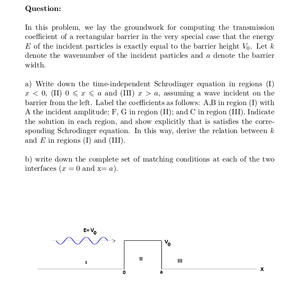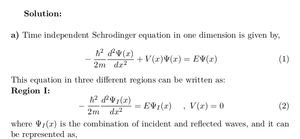Solved Problems in Physics, Physical Chemistry, and Computer Simulation Projects for College and Graduate Students.# Skimming a Barrier: Wave Function and Matching Conditions

Regular price
\$3.00
Sale price
\$3.00
Tax included.

Question:

In this problem, we lay the groundwork for computing the transmission coefficient of a rectangular barrier in the very special case that the energy E of the incident particles is exactly equal to the barrier height V0. Let k denote the wavenumber of the incident particles and a denote the barrier width.
a) Write down the time-independent Schrodinger equation in regions (I) x < 0, (II) 0 ≤ x ≤ a and (III) x > a, assuming a wave incident on the barrier from the left. Label the coefficients as follows: A,B in region (I) with A the incident amplitude; F, G in region (II); and C in region (III). Indicate the solution in each region, and show explicitly that is satisfies the corresponding Schrodinger equation. In this way, derive the relation between k and E in regions (I) and (III).
b) write down the complete set of matching conditions at each of the two interfaces (x = 0 and x= a).By purchasing this product, you will get the step by step solution of the above problem in pdf format and the corresponding latex file where you can edit the solution. I am also available to help  you with any possible question you may have.